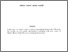# On the study of various equations concerning the Isoperimetric Theorems. Possible mathematical connections with some sectors of Number Theory, String Theory and some cosmological parameters

Nardelli, Michele and Nardelli, Antonio On the study of various equations concerning the Isoperimetric Theorems. Possible mathematical connections with some sectors of Number Theory, String Theory and some cosmological parameters. Number Theory and String Theory. (Unpublished)Preview
Text
Isoperimetric Theorems.pdf - Draft VersionView Item